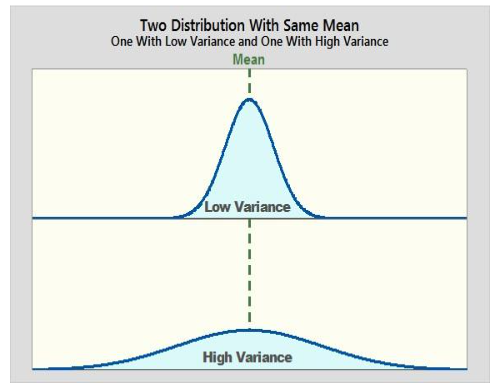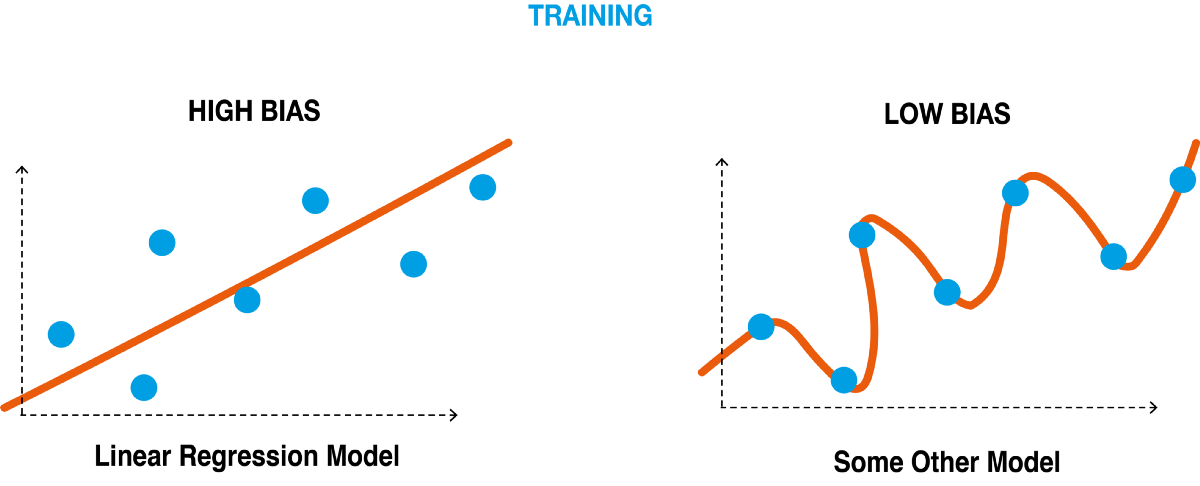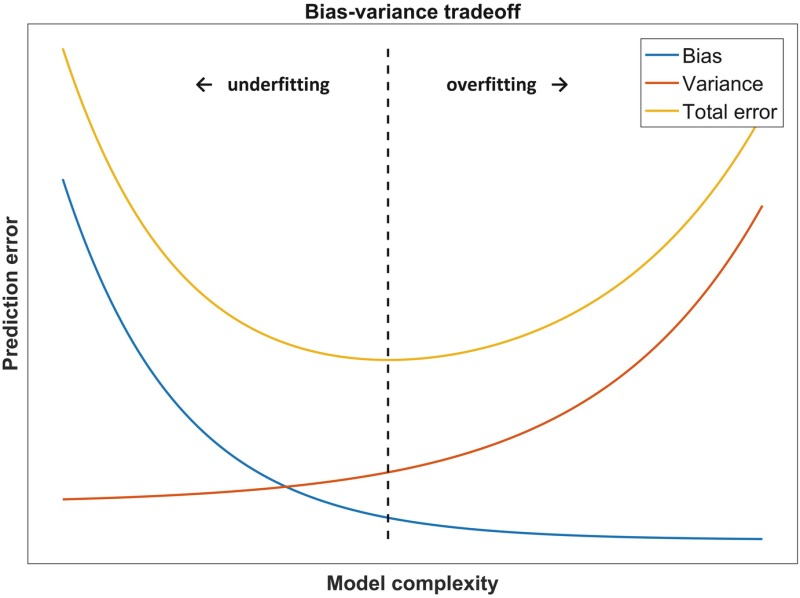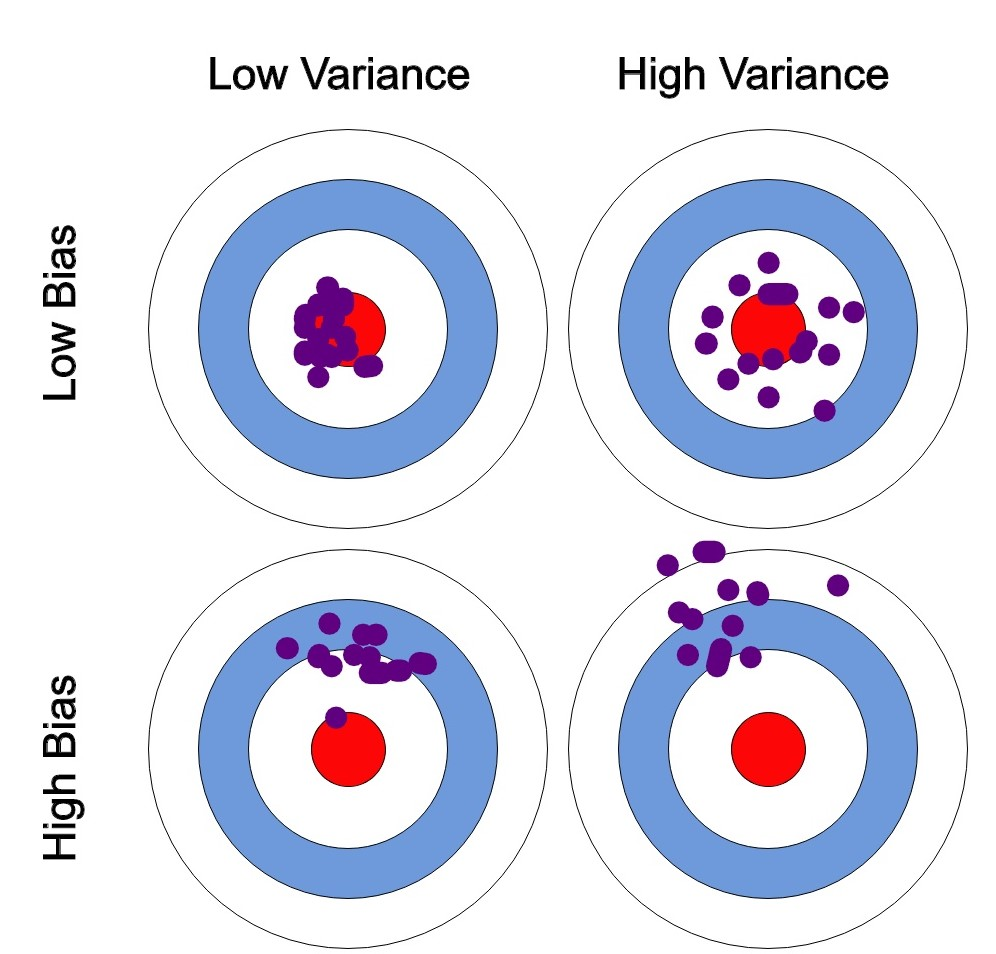# When developing machine learning models, everyone encounters two fundamental issues: underfitting and overfitting. If your model isn't performing as expected on input data, underfitting or overfitting is probably to blame.

The bias-variance tradeoff is crucial in data science when creating a machine learning model. Underfitting is seen when a model exhibits high bias. Overfitting is seen in the model when it shows high variance. Therefore, it's essential to balance these two and reduce errors.We can plainly observe locations with high bias and high variance in the above illustration that are both underfitting and overfitting the model.

The region where validation error and training error is almost the same is when Underfitting occurs and the region where validation error and training error greatly diverge is when Overfitting occurs.

To build accurate models we need to understand the difference between Bias and Variance. In this article, we will discuss Bias, Variance, and the trade-off between them.

## What is Bias?

Algorithmic bias sometimes referred to as Bias in an ML model, is the situation in which the model overvalues some features in an attempt to generalize the data, which results in a complex model.The difference between the average prediction of our model and the actual value that we are attempting to predict is used to measure it.

A high bias model value causes the model to be oversimplified, which increases inaccuracy in both training data and test data.

A low bias model value indicates that the model learned fewer things about the target feature which results in the inaccuracy of the model due to bias error.

## What is Variance?

As a measure of dispersion, Variance allows us to determine how widely apart a set of numbers is from their mean value.High variance-low Variance

In other words, a machine learning model's variance is nothing more than the variability of our model's prediction for a certain data point, which reveals the spread of the data.

High Variance indicates overfitting and a failure to generalize to previously unexplored data. On training data set, this leads to good accuracy, but on unseen data, it leads to significant inaccuracy due to variance error.

Low Variance indicates that the predicted data and actual data are closer to each other and prediction errors are low.

## How are bias and variance related?

Bias and Variance are inversely connected with each other. If the bias is high then we get a model with low variance. Similarly, if the bias is low, the model fits properly but it will lead to high variance. It is difficult to get a model that has low bias and low variance.

The case of having low variance and high bias reduces the risk of inaccurate predictions. But the model will not properly generalize the dataset.

Similarly, low bias and high variance will generalize the dataset but gives inaccurate predictions thereby increasing the complexity of the model.Relation between Bias and Variance

If we look at the above graph, we can clearly see that the model complexity is very less when there is a high bias but the error is very high. The model prediction seems to be affected at this point.

On the other side where there is low bias and high variance, the error is high, and hence it is not an accurate model. The training set is generalized well but the predictions are not very accurate.

The training dataset seems to be not generalizing well at both ends. There is a need to achieve low bias and get a less complex model. But we can’t achieve this just like that when the model is affected by bias and variance.

To generalize the model well and reduce the model complexity there is a need for a bias variance trade off where these two values are balanced in such a way that our model complexity is optimum and error is also minimum.

There are several ways to balance Bias and Variance. In the next section, we will discuss this bias variance trade off and the ways to tackle this bias variance tradeoff.

So far we have seen what is bias, variance, and the relation between them. To achieve optimum model complexity, and accurate predictions we need to find a point where the bias and variance are balanced with the total error of the model being at a minimum.If we look at the above graph, we can clearly see the predictions and actual values for different cases of bias and variance. The case of low bias and low variance is the ideal one but it is impossible to achieve that in practice.

The case of high variance, and low bias seems to be better than all the remaining cases where the actual values match with predicted values. There are different ways to tackle this bias-variance trade off.

One way is to increase the complexity of the model. This will decrease the bias and increase the variance. But as long as we keep the variance at an acceptable level we can get good accuracy on the training data. We can also get a good total error. The total error is the sum of variance error and bias error.

The other way is to increase the size of the training data set. When the model overfits, this method can be used. This also allows us to increase the complexity of the model without many variance errors.

But one thing that must be kept in mind is that increasing the training data set may not be fruitful when the model is a low bias one or the model underfits. Therefore this method can be used only when we have high variance situations.

## Conclusion

Bias and Variance are two important things that should be considered while building any machine learning model. The objective of any supervised learning algorithm is to minimize these two quantities. But it is not possible to do it in practice. It is due to the following reasons:

• Increasing the variance will always decrease bias.
• Decreasing the variance will always increase bias.

We need to keep the above two things in mind while building any machine learning model. We should not overfit or underfit our model. Most of the time either of these situations arises while building machine learning models.

Hence a trade-off where the bias is low and variance is at acceptable levels is to be done to get a model with minimum error. In this way, we can avoid overfitting and underfitting and get a good model that generalizes the training set.

Read More from Strictly By The Numbers

## Subscribe to Strictly Learning

### By Strictly By The Numbers

Tune into our e-learning platform to remain abreast with the latest Technological trends in Data Science, Cloud Engineering & Artificial Intelligence, curated by industry experts! Hands-on code tutorials and demos using real-life use cases.